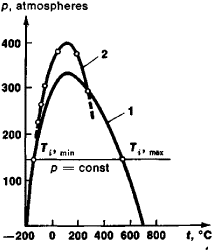# Inversion Curve

The following article is from The Great Soviet Encyclopedia (1979). It might be outdated or ideologically biased.

## Inversion Curve

a curve on a phase diagram that bounds the region of those phases (states) of a substance for which the transition of the substance from higher pressure to lower (in the process of throttling) is associated with a temperature drop. Beyond this region, the substance (a gas or liquid) heats on throttling. The constant-pressure line (isobar p = constant; see Figure 1) intersects the inversion curve at the two inversion points: the upper (Ti, max) and the lower (Ti, min). The upper point lies in the region of the gaseous state of the substance, and the lower in the liquid state.Figure 1. Inversion curves for air in pressure-temperature coordinates calculated according to (1) the Van der Waals equation and (2) experimental data

When Ti, min < T < Ti, max in a certain interval of pressure, the gas can be liquefied by throttling, but when T > Ti, max this cannot be accomplished. The closer a gas is in its properties to an ideal gas, the lower its inversion temperature (for hydrogen, for example, at atmospheric pressure Ti, max = −57°C, while for helium, Ti, max = −239°C). To liquefy helium by throttling, its temperature must first be reduced to −239°C.

IU. N. DROZHZHIN